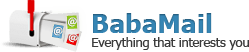# Ready For an Especially Tricky Math Test?

A+ A-
It seems to us, here at Baba-Mail, that our readers have become quite adept at math! So we cooked up this more challenging math test for you! It starts easy, but gets quite hard towards the end - can any of you get more than 10 out of these 12 questions? If so, we will be truly impressed!If Eric runs 4 miles in one hour, how long does it take him to run 18 miles?
4 hours
4.5 hours
5 hours
5.5 hoursWhich of these shapes has the most sides?
Hexagon
Octagon
Rhombus
TrapeziumWhat is 3/2 as a decimal?
1.15
1.1
1.5
3.2How many triangles is this cat made of?
The tail alone have 7 triangles, you must remember that a triangle cut at the tip always creates 2 triangles.
20
18
15
10A test has two papers. Amy scores 33 out of 60 on paper 1 and 75 out of 100 on paper 2. Work out the % score for the test
65.5 or 66
66.5 or 67
67.5 or 68
68.5 or 69A school has 720 boys and 700 girls. The probability that a boy chosen at random studies French is 2/3. The probability that a girl chosen at random studies French is 3/5 . Work out the number of students in the school who study French.
850
900
866
9753/5 of a number is 162. Work out the original number.
265
270
275
280Solve the simultaneous equations: 2X + Y = 18X - Y = 6
X = 4 and Y = 1
X = 0.6 Y= 4.8
X = 16 and Y = 4
X = 18 and Y = 2If the time is 10 and 15 minutes, how many degrees would there be between the hour and minute hands?
105 degrees
90 degrees
65 degrees
45 degreesIn a particular game, there can be eight players at one time on the pitch and they last for seventy minutes. In the total duration, six players substitute with each player. All the players are then for the same time on the pitch including the substitutes. Can you calculate the time each player was on the pitch?
The total number of players = 8 + 6 = 14 According to the question, only eight players can be present on the field. Thus calculating the total time: 70 * 8 = 560. Since we know there are 14 players we divide by 14 to get 40.
30 minutes
40 minutes
50 minutes
45 minutesBrian and Sam decided to play badminton against each other. They won't stop unless one of them won \$50. For every game, they bet \$10. If Brian won exactly 3 games, How many games did they play in total?
Brian won 3 games, so Sam had to win 3 (to balance 3 games he lost) + 5 games (required for min \$50) = 8 games Total Games = 3 + 8 = 11 games.
11
8
12
9To find the question mark number, you may need to think creatively... (there are two correct answers)
Solution A : Add the number of the last line to the current line 1 + 4 = 5 5 + 2 + 5 = 12 12 + 3 + 6 = 21 21 + 8 + 11 = 40 Solution B: 1 + (1 x 4) = 5 2 + (2 x 5) = 12 3 + (3 x 6) = 21 8 + (8 x 11) = 96
40
96
32
19
54
70
This Test Proved a Bit Hard For YouMaybe this was a bit too hard for you, but as we meant for this to be challenging, there's no shame in that! Have a look at what you got wrong, and take some of our other math quizzes (we have a lot!) for some fun practice!
You Did Quite Well, But Didn't Beat the TestThat wasn't bad at all and in fact you got half or more of the questions right. That's no easy feat, but you're still some ways away from being our math winner in this quiz. Look at your mistakes and try to figure out what kept you from beating this math quiz.
You Crazy Son of a Gun, You Did It!We're floored! You must have an excellent relationship with math or you're some kind of ex math teacher. We're on to you! But seriously, we're so proud we could burst! Keep up the smarts!